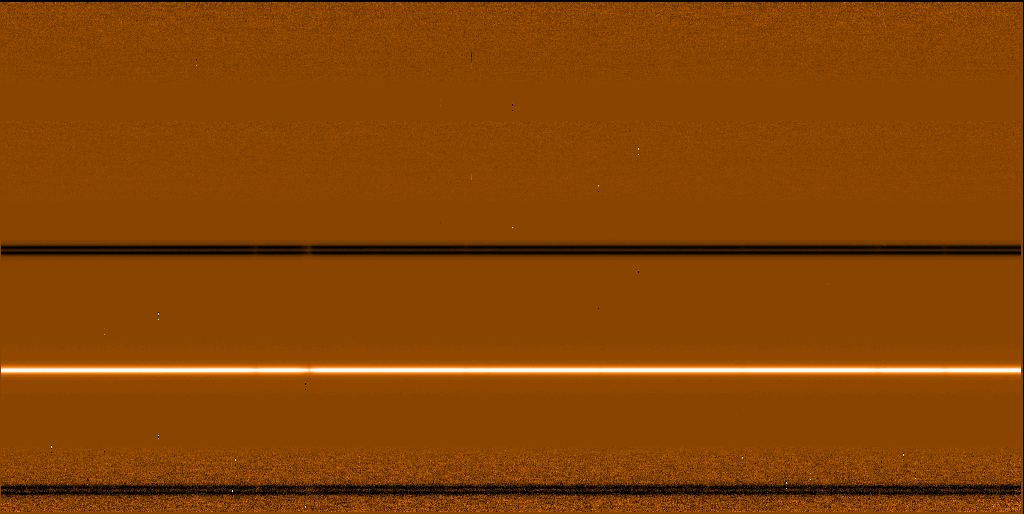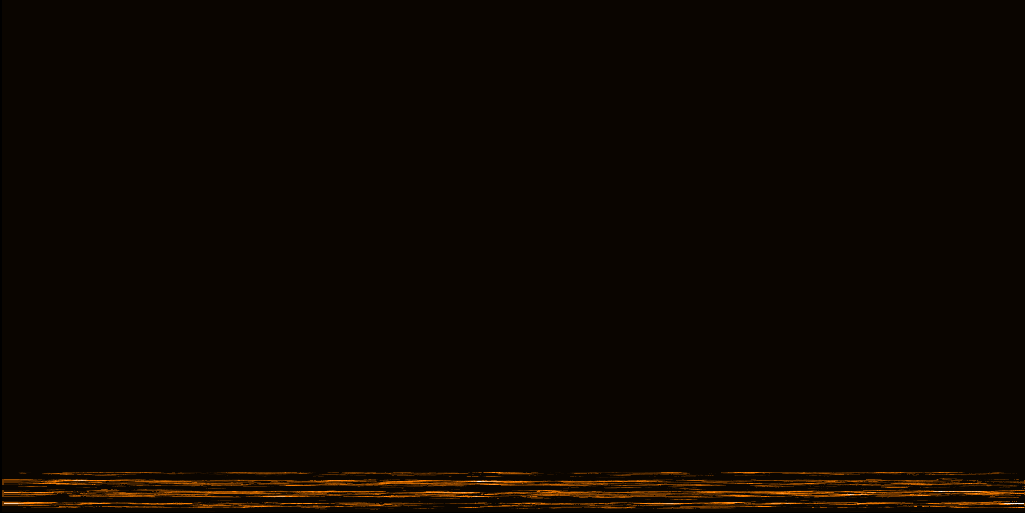CRIRES pipeline:
science recipe
 HOME INDEX SEARCH HELP NEWS

QUALITY CONTROL     HOME CAL | HC | refs | QCCRIRES QC HOMETOCgeneral infotrending & QC1features & problemsCRIRES Health Checksgeneral infoscience datascience recipecalib datacalib recipesassoc mapproduct namesgeneral infoCRIRES infogeneral infoCRIRES infoQC homeData ProductscalCheckerHealthChecksReference FramesQC1 databaseParanal autrep database(ESO internal) [best viewing with style sheets and javascript enabled]Science data and recipe

SCIENCE frames (crires_spec_jitter)
DPR.CATG = SCIENCE, DPR.TYPE = OBJECT, DPR.TECH = SPECTRUM,DIRECT,OTHER or
DPR.CATG = SCIENCE, DPR.TYPE = OBJECT, DPR.TECH = SPECTRUM,DIRECT,JITTER or
DPR.CATG = SCIENCE, DPR.TYPE = OBJECT, DPR.TECH = SPECTRUM,NODDING,OTHER or
DPR.CATG = SCIENCE, DPR.TYPE = OBJECT, DPR.TECH = SPECTRUM,NODDING,JITTERRaw science frame (at one nodding position)

Recipe. The pipeline recipe crires_spec_jitter performs the full reduction of science and standard star frames. The following steps are applied:

• dark subtraction
• correction for detector non-linearity
• flat-fielding
• combination of nodding exposures
• spectrum extraction
• wavelength calibration
• calculation of instrument throughput, etc. (spectro-photometric standards only).

Dark subtraction. Dark subtraction is performed on each input raw frame if a master dark (PRO.CATG = CALPRO_DARK) is provided. This is usually only needed for DIRECT observations (no nodding).

Non-linearity. Correction for non-linearity is performed if the coefficient images from detmon_ir_lg are provided (PRO.CATG COEFFS_CUBE, which has the A, B, and C images). That recipe uses a series of flats with increasing exposure level and fits the function

signal = A + B * DIT + C * DIT^2

for each pixel. Assuming that the detector is linear for small exposure levels, then the first derivative of the measured and the true signal are the same. Together with signal_true(DIT=0) = 0, this gives signal_true = B * DIT. Insertion into the equation above results in

signal = A + signal_true + C/B^2 * signal_true^2.

Finally, this gives the correction for each pixel:

signal_true = (-B^2 + B^2 * sqrt(1 - 4*C*(A-signal)/B^2)) / (2*C).

Flat-fielding. The input raw frames are divided by a (normalised) flat field.

Bad pixel correction. If a bad pixel map is provided, bad pixel are replaced by the average of their neighbours.

Image combination. In case of nodding observations, the individual exposures are combined after dark subtraction, correction for non-linearity, and flat-fielding have been applied. The combined image is used for the spectrum extraction and is saved as a separate output product.Example combined frame

Spectrum extraction. Two different methods are applied: rectangular and optimal extraction. Both use a weighting function. In case of rectangular extraction, the function is constant over N pixels which gives a simple average across the slit. For optimal extraction, the spectral profile is fitted. Each weighting function is normalised so that the integral over it gives 1. The normalisation factors are given in the output product. (In case of rectangular extraction, the normalisation factor is constant and equal to N.) The extracted spectra from both methods are contained in the output product. The weighting profile for optimal extraction is saved in a separate product and can be used to verify that the spectrum has been correctly identified and traced.Example of a good profile imageExample of a bad profile image where the spectrum could not be traced due to a low signal

Wavelength calibration. If a wavelength solution from an arc lamp or gas cell exposure is present then this result is applied for the wavelength calibration of the science data. Otherwise, the wavelength calibration is obtained from sky lines present in the science exposure. The latter is usually the case for settings above 2500 nm.

The recipe also calls the physical model which gives an alternative wavelength calibration based on the state of the instrument. The model results are added as an additional column in the output product. The wavelength maps from both the physical model and the solution based on measured lines are provided as separate products and can be compared with each other. The physical model gives a uniform solution for all four detectors but the solution can have an offset compared to the line solution since actual line positions are not taken into account. For several settings, there are currently also problems with the model computation which result in incomplete wavelength maps.

Products. The following science products are created by the pipeline:

 product category (PRO CATG)* product index** product code*** delivered? format comments EXTRACT_WL_TAB EXTRACT_SENS_TAB EXTRACT_TELLURIC_TAB 0000 SEXT yes table main product with extracted spectrum COMBINED_IMA 0001 SCOM yes 2D combined image of nodding positions CONTRIBUTION_IMA 0002 SCON yes 2D contribution map to combined image PROFILE_IMA 0003 SPRF yes 2D weighting profile used in optimal extraction BGD_MAP_IMA 0004 SBGD yes 2D map used for background noise calculation WL_MAP_IMA 0005 SWMA yes 2D wavelength map from the solution applied for wavelength calibration WL_MAP_MODEL_IMA 0006 SWMP yes 2D wavelength map from the model
* coded as HIERARCH.ESO.PRO.CATG in the fits header
** index of the PIPEFILE name, coded as PIPEFILE in the fits header
*** used in the delivered name

Data format. All products come as FITS files with 4 extensions which contain the pipeline results (either table or pixel data) for each of the 4 arrays of the detector. The main product (EXTRACT_WL_TAB in case of science data, EXTRACT_SENS_TAB or EXTRACT_TELLURIC_TAB in case of a standard star) has the following table columns:

 column name description Extracted_RECT extracted spectrum (average/rectangular extraction) Extracted_OPT extracted spectrum (optimal extraction) Error_RECT error from rectangular extraction Error_OPT error from optimal extraction Weight_Norm_RECT normalisation factor (rectangular extraction) Weight_Norm_OPT normalisation factor for extraction profile (optimal extraction) Background_Noise noise used for error calculation Wavelength wavelength from cross-correlation method Wavelength_model wavelength from physical modelSend comments to   Last update: July 7, 2011